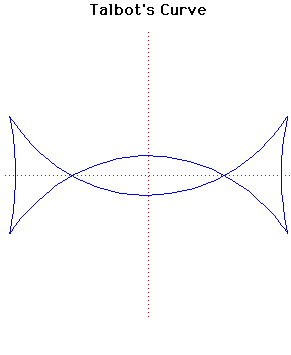# Curves

### Talbot's CurveParametric Cartesian equation:
$x = (a^{2} + f^{2}\sin^{2}(t))\cos(t)/a, y = (a^{2} - 2f^{2} + f^{2}\sin^{2}(t))\sin(t)/b$

### Description

This curve was investigated by Talbot.

Talbot's curve is the negative pedal of an ellipse with respect to its centre. It has four cusps and two nodes provided the square of the eccentricity of the ellipse is greater than $\large\frac{1}{2}\normalsize$.

### Associated Curves

Definitions of the Associated curves